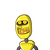# If alpha and beta are the zeros of polynomial 3 x^2-5x-9 find value of 2/ Alpha + 2/ beta.. Please solve this question fast, don’t

If alpha and beta are the zeros of polynomial 3 x^2-5x-9 find value of 2/ Alpha + 2/ beta.. Please solve this question fast, don’t post any irrelevant answer.. Class 10 maths ​

### 1 thought on “If alpha and beta are the zeros of polynomial 3 x^2-5x-9 find value of 2/ Alpha + 2/ beta.. Please solve this question fast, don’t”

1.$$\frac{ – 10}{9}$$

Step-by-step explanation:

By cross multiplying, we get

$$\frac{2}{ \alpha } + \frac{2}{ \beta } = \frac{2( \alpha + \beta) }{ \alpha \beta }$$

Now, alpha and beta are zeros of quadratic x^2-5x-9

so using the property for sum of roots and product of roots of a quadratic polynomial, we get

$$\alpha + \beta = \frac{ – ( – 5)}{1} = 5$$

$$\alpha \beta = \frac{ – 9}{1} = – 9$$

So,

$$\frac{2}{ \alpha } + \frac{2}{ \beta } = \frac{2( \alpha + \beta) }{ \alpha \beta } = \frac{2 \times 5}{ – 9} = \frac{ – 10}{9}$$